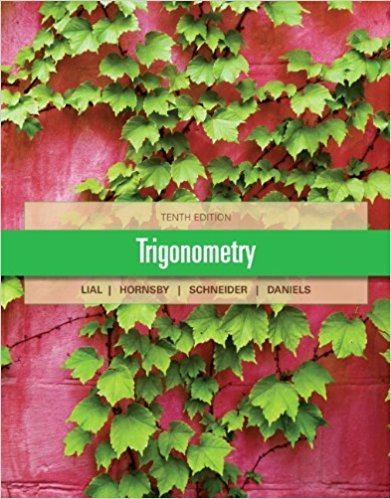×
×

# Solutions for Chapter 6.4: Equations Involving Inverse Trigonometric Functions## Full solutions for Trigonometry | 10th Edition

ISBN: 9780321671776Solutions for Chapter 6.4: Equations Involving Inverse Trigonometric Functions

Solutions for Chapter 6.4
4 5 0 261 Reviews
17
2
##### ISBN: 9780321671776

This expansive textbook survival guide covers the following chapters and their solutions. Since 54 problems in chapter 6.4: Equations Involving Inverse Trigonometric Functions have been answered, more than 34243 students have viewed full step-by-step solutions from this chapter. Trigonometry was written by and is associated to the ISBN: 9780321671776. Chapter 6.4: Equations Involving Inverse Trigonometric Functions includes 54 full step-by-step solutions. This textbook survival guide was created for the textbook: Trigonometry, edition: 10.

Key Math Terms and definitions covered in this textbook
• Dimension of vector space

dim(V) = number of vectors in any basis for V.

• Echelon matrix U.

The first nonzero entry (the pivot) in each row comes in a later column than the pivot in the previous row. All zero rows come last.

• Exponential eAt = I + At + (At)2 12! + ...

has derivative AeAt; eAt u(O) solves u' = Au.

• Free variable Xi.

Column i has no pivot in elimination. We can give the n - r free variables any values, then Ax = b determines the r pivot variables (if solvable!).

• Full row rank r = m.

Independent rows, at least one solution to Ax = b, column space is all of Rm. Full rank means full column rank or full row rank.

• Hankel matrix H.

Constant along each antidiagonal; hij depends on i + j.

• Kirchhoff's Laws.

Current Law: net current (in minus out) is zero at each node. Voltage Law: Potential differences (voltage drops) add to zero around any closed loop.

• Krylov subspace Kj(A, b).

The subspace spanned by b, Ab, ... , Aj-Ib. Numerical methods approximate A -I b by x j with residual b - Ax j in this subspace. A good basis for K j requires only multiplication by A at each step.

• Left inverse A+.

If A has full column rank n, then A+ = (AT A)-I AT has A+ A = In.

• Matrix multiplication AB.

The i, j entry of AB is (row i of A)·(column j of B) = L aikbkj. By columns: Column j of AB = A times column j of B. By rows: row i of A multiplies B. Columns times rows: AB = sum of (column k)(row k). All these equivalent definitions come from the rule that A B times x equals A times B x .

• Orthonormal vectors q 1 , ... , q n·

Dot products are q T q j = 0 if i =1= j and q T q i = 1. The matrix Q with these orthonormal columns has Q T Q = I. If m = n then Q T = Q -1 and q 1 ' ... , q n is an orthonormal basis for Rn : every v = L (v T q j )q j •

• Pivot.

The diagonal entry (first nonzero) at the time when a row is used in elimination.

• Rayleigh quotient q (x) = X T Ax I x T x for symmetric A: Amin < q (x) < Amax.

Those extremes are reached at the eigenvectors x for Amin(A) and Amax(A).

• Reduced row echelon form R = rref(A).

Pivots = 1; zeros above and below pivots; the r nonzero rows of R give a basis for the row space of A.

• Schur complement S, D - C A -} B.

Appears in block elimination on [~ g ].

• Schwarz inequality

Iv·wl < IIvll IIwll.Then IvTAwl2 < (vT Av)(wT Aw) for pos def A.

• Simplex method for linear programming.

The minimum cost vector x * is found by moving from comer to lower cost comer along the edges of the feasible set (where the constraints Ax = b and x > 0 are satisfied). Minimum cost at a comer!

• Special solutions to As = O.

One free variable is Si = 1, other free variables = o.

• Toeplitz matrix.

Constant down each diagonal = time-invariant (shift-invariant) filter.

• Vandermonde matrix V.

V c = b gives coefficients of p(x) = Co + ... + Cn_IXn- 1 with P(Xi) = bi. Vij = (Xi)j-I and det V = product of (Xk - Xi) for k > i.

×